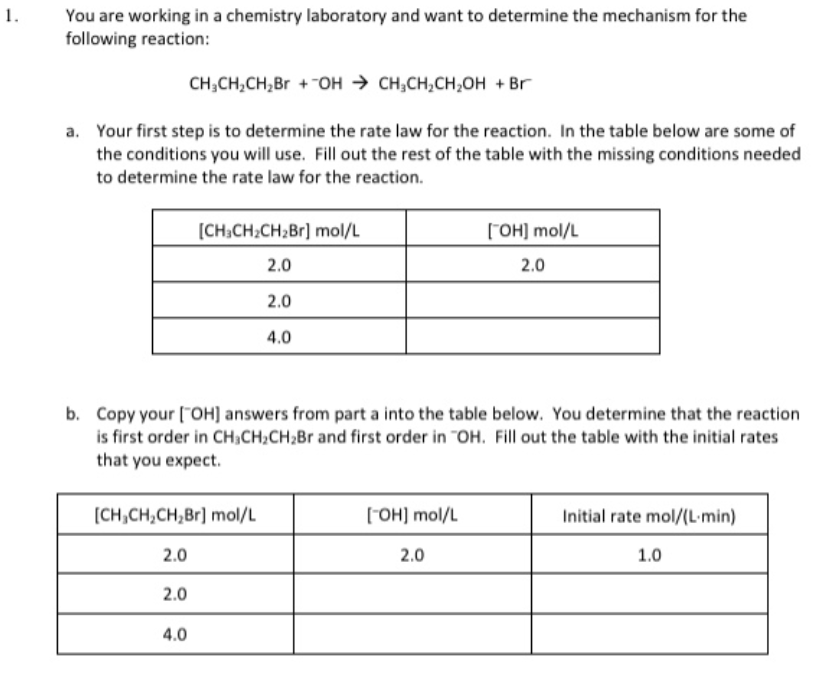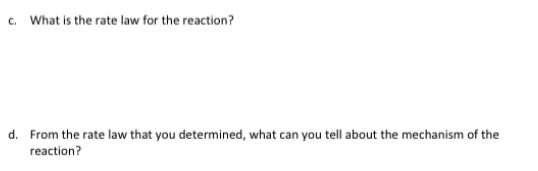# 1. You are working in a chemistry laboratory and want to determine the mechanism for the following reaction: CH3CH2CH2Br + -OH → CH3CH2CH2OH + Br^- a. Your first step is to determine the rate law for the reaction. In the table below are some of the conditions you will use. Fill out the rest of the table with the missing conditions needed to determine the rate law for the reaction. b. Copy your [-OH] answers from part a into the table below. You determine that the reaction is first order in CH3CH2CH2Br and first order in -OH. Fill out the table with the initial rates that you expect. c. What is the rate law for the reaction? d. From the rate law that you determined, what can you tell about the mechanism of the reaction?